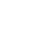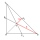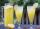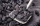Fractions - math word problems - page 21

1. Adding mixed numbersAdd this two mixed numbers: 1 5/6 + 2 2/11=
2. STRESSED wordEach letter in STRESSED is printed on identical cards, one letter per card and assembled in random order. Calculate the probability that the cards spell DESSERTS when assembled.
3. Unknown numberI think the number. I'll reduce it to its one-third. The result is then increased by one-third, and I get the number 12.
4. A manA man spends 5/9 of his money on rent, and 5/16 of the remainder on electricity. If the final balance remaining is 550 find how much was spent on rent
5. Water 31Richard takes 3 1/6 liters of water before noon and 2 3/5 liters of water after noon. How many litres of water does Richard consume a day ?
6. AndreAndre, Thomas, and Ivan split 88 postage stamps in a 2:5:4 ratio. How much did Thomas get?
7. RegroupingSubtract mixed number with regrouping: 11 17/20- 6 19/20
8. A largeA large gear will be used to turn a smaller gear. The large gear will make 75 revolutions per minute. The smaller gear must make 384 revolutions per minute. Find the smallest number of teeth each gear could have. [Hint: Use either GCF or LCM. ]
9. Medians 2:1Median to side b (tb) in triangle ABC is 12 cm long. a. What is the distance of the center of gravity T from the vertex B? b, Find the distance between T and the side b.
10. Addition of mixed numeralsAdd two mixed fractions: 2 4/6 + 1 3/6How many 1/4 cup servings are in 2 and 1/3 cups of lemonade?
12. DivideDivide area of rectangles with dimensions 32m and 10m by the ratio 7: 9. What area corresponds to a smaller section?
13. Ratio of volumesIf the heights of two cylindrical drums are in the ratio 7:8 and their base radii are in the ratio 4:3. What is the ratio of their volumes?
14. Adding mixed numerals3 3/4 + 2 3/5 + 5 1/2 Show your solution.
15. Report 2A School reports students to teacher ratio of 6:1. If there are 45 teachers in the School, how many students are there?
16. Bitoo and ReenaBitoo ate 3/5 part of an apple and the remaining part was eaten by his sister Reena. How much part of an apple did Renna eat? Who had the larger share? By how much?
17. Coal mineThe monthly plan of 17,000 tons of coal exceeded the mine by 1/25. How many tonnes of coal have been harvested from the mine above plan?
18. Tallest and shortestJenn is the tallest on the team. He is 1 1/2 times as tall as the shortest girl whom is 4 1/4 feet tall. How tall is Jebb?
19. Profit growthThe profit of a company increased by 25% during the year 1992, increased by 40% during the year 1993, decreased by 20% in the year 1994 and increased by 10% during the year 1995. Find the average growth in the profit level over the four years periods?
20. EricaErica bought 3 1/2 yards of fabric. If she uses 2/3 of the fabric, how much will she have left?

Do you have an interesting mathematical word problem that you can't solve it? Enter it, and we can try to solve it.

To this e-mail address, we will reply solution; solved examples are also published here. Please enter e-mail correctly and check whether you don't have a full mailbox.

Need help calculate sum, simplify or multiply fractions? Try our fraction calculator.